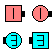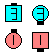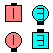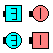Definitions of Square Dance Calls and Concepts
Single Checkmate [C3A]Index -->  Plus  |  A1  |  A2  |  C1  |  C2  |  C3A  |  C3B  |  C4  |  NOL  |
Definitions (Text Only) -->  Plus  |  A1  |  A2  |  C1  |  C2  |  C3A  |  C3B  |  C4  |  NOL  |
 Find call:

Single Checkmate -- [C3A]
(author unknown)
 C3A:\$B8@8l(BFrom a 2 x 2. EN: 10
2 x 2 \$B\$+\$i!%(B JP: 10

Leaders Box Circulate twice and 1/4 In (Box Transfer) as Trailers Box Circulate, 1/4 In, and Box Circulate. EN: 20
Leaders \$B\$O(B Box Circulate twice \$B\$r\$7\$F(B 1/4 In (Box Transfer) \$B\$r(B, Trailers \$B\$O(B Box Circulate - 1/4 In - Box Circulate \$B\$r\$7\$^\$9!%(B JP: 20

Ends in a 2 x 2. EN: 30
2 x 2 \$B\$G=*\$o\$j\$^\$9!%(B JP: 30Single Checkmate\$B\$NA0(B \$B8e(BSingle Checkmate\$B\$NA0(B \$B8e(B

\$BCm

• Original Trailers can not Roll. Single Checkmate is the Single version of Checkmate The Column [A2]. Note that on Checkmate The Column, the Trailing two cannot Roll since their last part is to Step Ahead. EN: 40
\$B%*%j%8%J%k\$N(B Trailers \$B\$O(B Roll \$B\$G\$-\$^\$;\$s!%(B Single Checkmate \$B\$O(B Checkmate The Column [A2] \$B\$N(B Single \$B\$N%P!<%8%g%s\$G\$9!%(B Checkmate The Column \$B\$K\$D\$\$\$F\$b(B, Trailing two \$B\$O:G8e\$N%Q!<%H\$,(B Step Ahead \$B\$J\$N\$G(B, Roll \$B\$G\$-\$^\$;\$s!%(B JP: 40
• Single Checkmate has historically been known as Split Checkmate when done on each side and Box Checkmate when done in the center or from a Box. EN: 50
Single Checkmate \$B\$O(B \$BNr;KE*\$K(B, each side \$B\$G9T\$&\$H\$-\$O(B Split Checkmate \$B\$H%3!<%k\$5\$l(B, center \$B\$"\$k\$\$\$O(B box \$B\$G9T\$&\$H\$-\$O(B Box Checkmate \$B\$H%3!<%k\$5\$l\$F\$-\$^\$7\$?!%(B JP: 50
• Cheat: Box Counter Rotate 1/4; Box Circulate. This cheat emphasizes that only the original Leaders can Roll. Some other glossaries list a similar cheat (Box Circulate; Box Counter Rotate 1/4) which makes it seem as if everyone can Roll. EN: 60
Cheat: Box Counter Rotate 1/4 \$B\$r\$7\$F(B, Box Circulate \$B\$G\$9!%(B \$B\$3\$N(B cheat \$B\$O(B, \$B%*%j%8%J%k\$N(B Leaders \$B\$@\$1\$,(B Roll \$B\$G\$-\$k\$H\$\$\$&\$3\$H\$r\$O\$C\$-\$j\$5\$;\$^\$9!%B>\$N;qNA\$K\$O(B, \$BF1\$8\$h\$&\$J(B cheat (Box Circulate; Box Counter Rotate 1/4) \$B\$,\$"\$j\$^\$9\$,(B, \$B\$=\$l\$G\$OA40w\$,(B Roll \$B\$G\$-\$k\$h\$&\$K;W\$(\$F\$7\$^\$\$\$^\$9!%(B JP: 60

Checkmate The Column [A2] (H.P. Williams 1975):
From Columns. First Two Circulate 4 spots & 1/4 In as Last Two Circulate twice, 1/4 In, & Circulate. Normal Columns end in Parallel Two-Faced Lines. EN: 295
Column \$B\$+\$i!%(B \$B;O\$a\$N(B 2 \$B?M\$O(B Circulate 4 spots \$B\$r\$7(B 1/4 In \$B\$r(B, \$B8e\$N(B2\$B?M\$O(B Circulate twice - 1/4 In - Circulate \$B\$r\$7\$^\$9!%(B \$BIaDL\$N(B Column \$B\$+\$i\$O(B, \$BJ?9T\$J(B Two-Faced Line \$B\$G=*\$o\$j\$^\$9!%(B JP: 295

\$BSingle CONCEPT [C3A,C3B,C4].CALLERLAB definition for Single CheckmateChoreography for Single CheckmateComments? Questions? Suggestions?

 C3A: